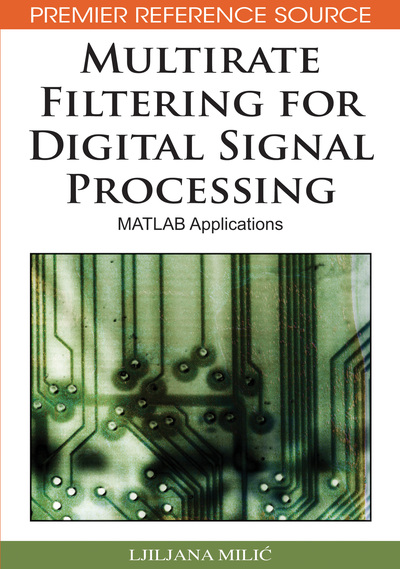# Multirate Techniques in Filter Design and Implementation

Ljiljana Milic (Mihajlo Pupin Institute, Serbia)
DOI: 10.4018/978-1-60566-178-0.ch009
Available
\$33.75
List Price: \$37.50
10% Discount:-\$3.75
TOTAL SAVINGS: \$3.75

## Abstract

Digital filters with sharp transition bands are difficult, sometimes impossible, to be implemented using single-stage structures. A serious problem with a single-stage sharp FIR filter is its complexity. The FIR filter length is inversely proportional to the transition–width and complexity becomes prohibitively high for sharp filters, (Lim, 1986). IIR filters with sharp transition bands suffer from extremely high sensitivities of transfer function poles. In many practical cases, the multirate approach is the promising solution that could be applied for implementation of a sharp FIR or IIR filter. In this chapter, we present two methods for designing filters having narrow transition bandwidths: multistage filtering suitable for narrowband filters, and the method based on multirate and complementary filtering, which may be used for filters of arbitrary bandwidths.
Chapter Preview
Top

## Solving Complex Filtering Problems Using Multirate Techniques

Multirate techniques provide efficient tools for constructing digital filters with equal input and output sampling rates. Multirate techniques are shown to be an appropriate solution for the specifications that require a single-rate filter of a very high order. Instead of designing the single-rate filter, one can achieve a more effective solution with a multirate filter constructed as a combination of several low-order subfilters and sampling rate alteration devices. The benefits that can be achieved in comparison with the single-rate design are threefold:

• 1.

The overall filtering problem can be shared between several lower-order subfilters.

• 2.

The final wordlength effects on the overall filter performances are considerably reduced.

• 3.

The arithmetic operations in subfilters can be evaluated at the reduced sampling rate thus reducing the overall computational complexity.

On the other hand, the multirate solutions introduce the unwanted effects that should be taken into account and minimized. As shown earlier in Chapter II, the signal distortions are produced when applying two basic operations of sampling rate conversion, down-sampling and up-sampling:

• 1.

Each down-sampler produces aliasing.

• 2.

Each up-sampler produces imaging.

Therefore, the subfilters in a multirate filter should sufficiently suppress the aliasing and imaging caused by all down-samplers and up-samplers that are incorporated in the multirate filtering structure.

The expressions that relate the z-transforms and the Fourier transforms of down-sampled and up-sampled signals with the z-transforms and the Fourier transforms of original signals have been shown earlier in Chapter II. Those expressions will also be used through this Chapter for the analysis of aliasing and imaging effects.

Let us denote with {x[n]} and X(z) the original signal and its z-transform, respectively. For the down-sampled-by-M signal {xD[n]}, the z-transform XD(z) is given by (see equation (2.17)),, (1) and, accordingly, the corresponding Fourier transform follows, equation (2.18),. (2)

The z-transform relation for up-sampling as given in equation (2.22), is expressed by,

(3) where with XI(z) we denote the z-transform of the up-sampled-by-L signal, {xI[n]}. The Fourier transform relations for up-sampler according to equation (2.23) is given by,. (4)

## Complete Chapter List

Search this Book:
Reset